## Posts Tagged ‘PID’

### Improving the Beginner’s PID: On/Off

Friday, April 15th, 2011

(This is Modification #5 in a larger series on writing a solid PID algorithm)

### The Problem

As nice as it is to have a PID controller, sometimes you don’t care what it has to say.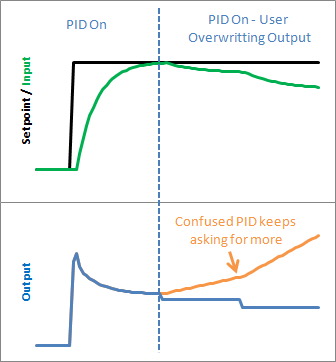Let’s say at some point in your program you want to force the output to a certain value (0 for example) you could certainly do this in the calling routine:

void loop()
{
Compute();
Output=0;
}

This way, no matter what the PID says, you just overwrite its value. This is a terrible idea in practice however. The PID will become very confused: “I keep moving the output, and nothing’s happening! What gives?! Let me move it some more.” As a result, when you stop over-writing the output and switch back to the PID, you will likely get a huge and immediate change in the output value.

### The Solution

The solution to this problem is to have a means to turn the PID off and on. The common terms for these states are “Manual” (I will adjust the value by hand) and “Automatic” (the PID will automatically adjust the output). Let’s see how this is done in code:

### The Code

```
/*working variables*/
unsigned long lastTime;
double Input, Output, Setpoint;
double ITerm, lastInput;
double kp, ki, kd;
int SampleTime = 1000; //1 sec
double outMin, outMax;
bool inAuto = false;

#define MANUAL 0
#define AUTOMATIC 1

void Compute()
{
if(!inAuto) return;
unsigned long now = millis();
int timeChange = (now - lastTime);
if(timeChange>=SampleTime)
{
/*Compute all the working error variables*/
double error = Setpoint - Input;
ITerm+= (ki * error);
if(ITerm> outMax) ITerm= outMax;
else if(ITerm< outMin) ITerm= outMin;
double dInput = (Input - lastInput);

/*Compute PID Output*/
Output = kp * error + ITerm- kd * dInput;
if(Output > outMax) Output = outMax;
else if(Output < outMin) Output = outMin;

/*Remember some variables for next time*/
lastInput = Input;
lastTime = now;
}
}

void SetTunings(double Kp, double Ki, double Kd)
{
double SampleTimeInSec = ((double)SampleTime)/1000;
kp = Kp;
ki = Ki * SampleTimeInSec;
kd = Kd / SampleTimeInSec;
}

void SetSampleTime(int NewSampleTime)
{
if (NewSampleTime > 0)
{
double ratio  = (double)NewSampleTime
/ (double)SampleTime;
ki *= ratio;
kd /= ratio;
SampleTime = (unsigned long)NewSampleTime;
}
}

void SetOutputLimits(double Min, double Max)
{
if(Min > Max) return;
outMin = Min;
outMax = Max;

if(Output > outMax) Output = outMax;
else if(Output < outMin) Output = outMin;

if(ITerm> outMax) ITerm= outMax;
else if(ITerm< outMin) ITerm= outMin;
}

void SetMode(int Mode)
{
inAuto = (Mode == AUTOMATIC);
}

```

A fairly simple solution. If you’re not in automatic mode, immediately leave the Compute function without adjusting the Output or any internal variables.

### The Result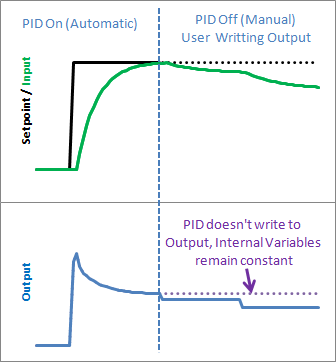It’s true that you could achieve a similar effect by just not calling Compute from the calling routine, but this solution keeps the workings of the PID contained, which is kind of what we need. By keeping things internal we can keep track of which mode were in, and more importantly it let’s us know when we change modes. That leads us to the next issue…
Next >>### Improving the Beginner’s PID: Reset Windup

Friday, April 15th, 2011

(This is Modification #4 in a larger series on writing a solid PID algorithm)

### The Problem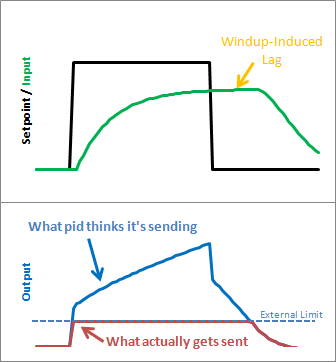Reset windup is a trap that probably claims more beginners than any other. It occurs when the PID thinks it can do something that it can’t. For example, the PWM output on an Arduino accepts values from 0-255. By default the PID doesn’t know this. If it thinks that 300-400-500 will work, it’s going to try those values expecting to get what it needs. Since in reality the value is clamped at 255 it’s just going to keep trying higher and higher numbers without getting anywhere.

The problem reveals itself in the form of weird lags. Above we can see that the output gets “wound up” WAY above the external limit. When the setpoint is dropped the output has to wind down before getting below that 255-line.

### The Solution – Step 1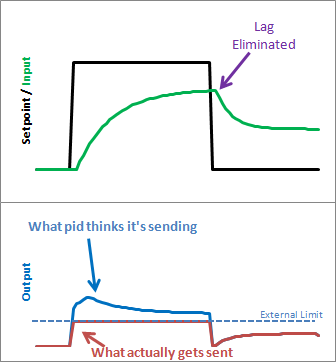There are several ways that windup can be mitigated, but the one that I chose was as follows: tell the PID what the output limits are. In the code below you’ll see there’s now a SetOuputLimits function. Once either limit is reached, the pid stops summing (integrating.) It knows there’s nothing to be done; Since the output doesn’t wind-up, we get an immediate response when the setpoint drops into a range where we can do something.

### The Solution – Step 2

Notice in the graph above though, that while we got rid that windup lag, we’re not all the way there. There’s still a difference between what the pid thinks it’s sending, and what’s being sent. Why? the Proportional Term and (to a lesser extent) the Derivative Term.

Even though the Integral Term has been safely clamped, P and D are still adding their two cents, yielding a result higher than the output limit. To my mind this is unacceptable. If the user calls a function called “SetOutputLimits” they’ve got to assume that that means “the output will stay within these values.” So for Step 2, we make that a valid assumption. In addition to clamping the I-Term, we clamp the Output value so that it stays where we’d expect it.

(Note: You might ask why we need to clamp both. If we’re going to do the output anyway, why clamp the Integral separately? If all we did was clamp the output, the Integral term would go back to growing and growing. Though the output would look nice during the step up, we’d see that telltale lag on the step down.)

### The Code

```/*working variables*/
unsigned long lastTime;
double Input, Output, Setpoint;
double ITerm, lastInput;
double kp, ki, kd;
int SampleTime = 1000; //1 sec
double outMin, outMax;
void Compute()
{
unsigned long now = millis();
int timeChange = (now - lastTime);
if(timeChange>=SampleTime)
{
/*Compute all the working error variables*/
double error = Setpoint - Input;
ITerm+= (ki * error);
if(ITerm> outMax) ITerm= outMax;
else if(ITerm< outMin) ITerm= outMin;
double dInput = (Input - lastInput);

/*Compute PID Output*/
Output = kp * error + ITerm- kd * dInput;
if(Output > outMax) Output = outMax;
else if(Output < outMin) Output = outMin;

/*Remember some variables for next time*/
lastInput = Input;
lastTime = now;
}
}

void SetTunings(double Kp, double Ki, double Kd)
{
double SampleTimeInSec = ((double)SampleTime)/1000;
kp = Kp;
ki = Ki * SampleTimeInSec;
kd = Kd / SampleTimeInSec;
}

void SetSampleTime(int NewSampleTime)
{
if (NewSampleTime > 0)
{
double ratio  = (double)NewSampleTime
/ (double)SampleTime;
ki *= ratio;
kd /= ratio;
SampleTime = (unsigned long)NewSampleTime;
}
}

void SetOutputLimits(double Min, double Max)
{
if(Min > Max) return;
outMin = Min;
outMax = Max;

if(Output > outMax) Output = outMax;
else if(Output < outMin) Output = outMin;

if(ITerm> outMax) ITerm= outMax;
else if(ITerm< outMin) ITerm= outMin;
}
```

A new function was added to allow the user to specify the output limits [lines 52-63]. And these limits are used to clamp both the I-Term [17-18] and the Output [23-24]

### The Result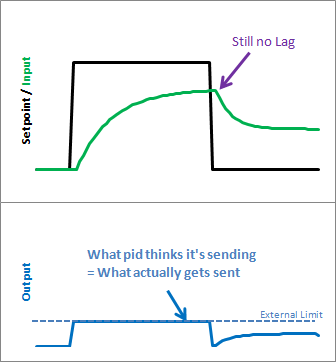As we can see, windup is eliminated. in addition, the output stays where we want it to. this means there’s no need for external clamping of the output. if you want it to range from 23 to 167, you can set those as the Output Limits.
Next >>### Improving the Beginner’s PID: Tuning Changes

Friday, April 15th, 2011

(This is Modification #3 in a larger series on writing a solid PID algorithm)

### The Problem

The ability to change tuning parameters while the system is running is a must for any respectable PID algorithm.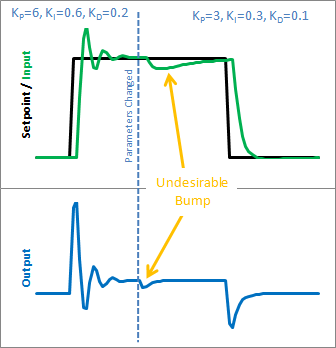The Beginner’s PID acts a little crazy if you try to change the tunings while it’s running. Let’s see why. Here is the state of the beginner’s PID before and after the parameter change above: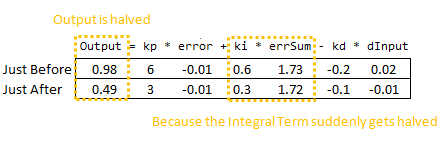So we can immediately blame this bump on the Integral Term (or “I Term”). It’s the only thing that changes drastically when the parameters change. Why did this happen? It has to do with the beginner’s interpretation of the Integral: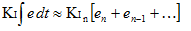This interpretation works fine until the Ki is changed. Then, all of a sudden, you multiply this new Ki times the entire error sum that you have accumulated. That’s not what we wanted! We only wanted to affect things moving forward!

### The Solution

There are a couple ways I know of to deal with this problem. The method I used in the last library was to rescale errSum. Ki doubled? Cut errSum in Half. That keeps the I Term from bumping, and it works. It’s kind of clunky though, and I’ve come up with something more elegant. (There’s no way I’m the first to have thought of this, but I did think of it on my own. That counts damnit!)

The solution requires a little basic algebra (or is it calculus?)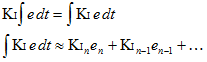Instead of having the Ki live outside the integral, we bring it inside. It looks like we haven’t done anything, but we’ll see that in practice this makes a big difference.

Now, we take the error and multiply it by whatever the Ki is at that time. We then store the sum of THAT. When the Ki changes, there’s no bump because all the old Ki’s are already “in the bank” so to speak. We get a smooth transfer with no additional math operations. It may make me a geek but I think that’s pretty sexy.

### The Code

```/*working variables*/
unsigned long lastTime;
double Input, Output, Setpoint;
double ITerm, lastInput;
double kp, ki, kd;
int SampleTime = 1000; //1 sec
void Compute()
{
unsigned long now = millis();
int timeChange = (now - lastTime);
if(timeChange>=SampleTime)
{
/*Compute all the working error variables*/
double error = Setpoint - Input;
ITerm += (ki * error);
double dInput = (Input - lastInput);

/*Compute PID Output*/
Output = kp * error + ITerm - kd * dInput;

/*Remember some variables for next time*/
lastInput = Input;
lastTime = now;
}
}

void SetTunings(double Kp, double Ki, double Kd)
{
double SampleTimeInSec = ((double)SampleTime)/1000;
kp = Kp;
ki = Ki * SampleTimeInSec;
kd = Kd / SampleTimeInSec;
}

void SetSampleTime(int NewSampleTime)
{
if (NewSampleTime > 0)
{
double ratio  = (double)NewSampleTime
/ (double)SampleTime;
ki *= ratio;
kd /= ratio;
SampleTime = (unsigned long)NewSampleTime;
}
}

```

So we replaced the errSum variable with a composite ITerm variable [Line 4]. It sums Ki*error, rather than just error [Line 15]. Also, because Ki is now buried in ITerm, it’s removed from the main PID calculation [Line 19].

### The Result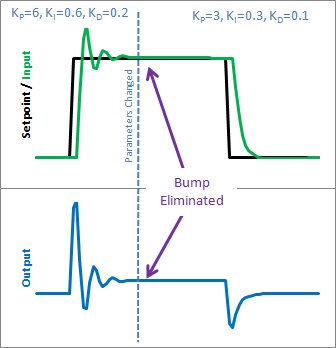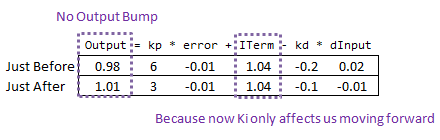So how does this fix things. Before when ki was changed, it rescaled the entire sum of the error; every error value we had seen. With this code, the previous error remains untouched, and the new ki only affects things moving forward, which is exactly what we want.
Next >>### Improving the Beginner’s PID – Derivative Kick

Friday, April 15th, 2011

(This is Modification #2 in a larger series on writing a solid PID algorithm)

### The Problem

This modification is going to tweak the derivative term a bit. The goal is to eliminate a phenomenon known as “Derivative Kick”.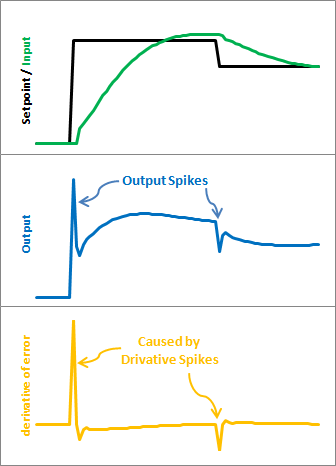The image above illustrates the problem. Since error=Setpoint-Input, any change in Setpoint causes an instantaneous change in error. The derivative of this change is infinity (in practice, since dt isn’t 0 it just winds up being a really big number.) This number gets fed into the pid equation, which results in an undesirable spike in the output. Luckily there is an easy way to get rid of this.

### The Solution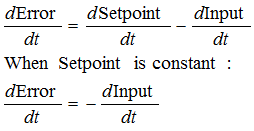It turns out that the derivative of the Error is equal to negative derivative of Input, EXCEPT when the Setpoint is changing. This winds up being a perfect solution. Instead of adding (Kd * derivative of Error), we subtract (Kd * derivative of Input). This is known as using “Derivative on Measurement”

### The Code

```/*working variables*/
unsigned long lastTime;
double Input, Output, Setpoint;
double errSum, lastInput;
double kp, ki, kd;
int SampleTime = 1000; //1 sec
void Compute()
{
unsigned long now = millis();
int timeChange = (now - lastTime);
if(timeChange>=SampleTime)
{
/*Compute all the working error variables*/
double error = Setpoint - Input;
errSum += error;
double dInput = (Input - lastInput);

/*Compute PID Output*/
Output = kp * error + ki * errSum - kd * dInput;

/*Remember some variables for next time*/
lastInput = Input;
lastTime = now;
}
}

void SetTunings(double Kp, double Ki, double Kd)
{
double SampleTimeInSec = ((double)SampleTime)/1000;
kp = Kp;
ki = Ki * SampleTimeInSec;
kd = Kd / SampleTimeInSec;
}

void SetSampleTime(int NewSampleTime)
{
if (NewSampleTime > 0)
{
double ratio  = (double)NewSampleTime
/ (double)SampleTime;
ki *= ratio;
kd /= ratio;
SampleTime = (unsigned long)NewSampleTime;
}
}
```

The modifications here are pretty easy. We’re replacing +dError with -dInput. Instead of remembering the lastError, we now remember the lastInput

### The Result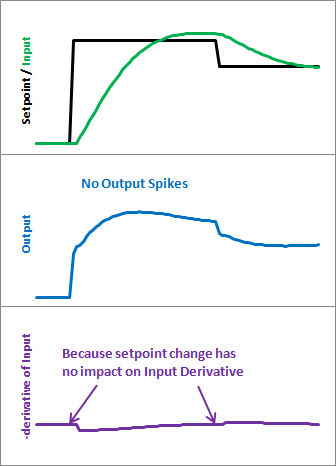Here’s what those modifications get us. Notice that the input still looks about the same. So we get the same performance, but we don’t send out a huge Output spike every time the Setpoint changes.

This may or may not be a big deal. It all depends on how sensitive your application is to output spikes. The way I see it though, it doesn’t take any more work to do it without kicking so why not do things right?
Next >>### Improving the Beginner’s PID – Sample Time

Friday, April 15th, 2011

(This is Modification #1 in a larger series on writing a solid PID algorithm)

### The Problem

The Beginner’s PID is designed to be called irregularly. This causes 2 issues:

• You don’t get consistent behavior from the PID, since sometimes it’s called frequently and sometimes it’s not.
• You need to do extra math computing the derivative and integral, since they’re both dependent on the change in time.

### The Solution

Ensure that the PID is called at a regular interval. The way I’ve decided to do this is to specify that the compute function get called every cycle. based on a pre-determined Sample Time, the PID decides if it should compute or return immediately.

Once we know that the PID is being evaluated at a constant interval, the derivative and integral calculations can also be simplified. Bonus!

### The Code

```/*working variables*/
unsigned long lastTime;
double Input, Output, Setpoint;
double errSum, lastErr;
double kp, ki, kd;
int SampleTime = 1000; //1 sec
void Compute()
{
unsigned long now = millis();
int timeChange = (now - lastTime);
if(timeChange>=SampleTime)
{
/*Compute all the working error variables*/
double error = Setpoint - Input;
errSum += error;
double dErr = (error - lastErr);

/*Compute PID Output*/
Output = kp * error + ki * errSum + kd * dErr;

/*Remember some variables for next time*/
lastErr = error;
lastTime = now;
}
}

void SetTunings(double Kp, double Ki, double Kd)
{
double SampleTimeInSec = ((double)SampleTime)/1000;
kp = Kp;
ki = Ki * SampleTimeInSec;
kd = Kd / SampleTimeInSec;
}

void SetSampleTime(int NewSampleTime)
{
if (NewSampleTime > 0)
{
double ratio  = (double)NewSampleTime
/ (double)SampleTime;
ki *= ratio;
kd /= ratio;
SampleTime = (unsigned long)NewSampleTime;
}
}
```

On lines 10&11, the algorithm now decides for itself if it’s time to calculate. Also, because we now KNOW that it’s going to be the same time between samples, we don’t need to constantly multiply by time change. We can merely adjust the Ki and Kd appropriately (lines 31 & 32) and result is mathematically equivalent, but more efficient.

one little wrinkle with doing it this way though though. if the user decides to change the sample time during operation, the Ki and Kd will need to be re-tweaked to reflect this new change. that’s what lines 39-42 are all about.

Also Note that I convert the sample time to Seconds on line 29. Strictly speaking this isn’t necessary, but allows the user to enter Ki and Kd in units of 1/sec and s, rather than 1/mS and mS.

### The Results

the changes above do 3 things for us

1. Regardless of how frequently Compute() is called, the PID algorithm will be evaluated at a regular interval [Line 11]
2. Because of the time subtraction [Line 10] there will be no issues when millis() wraps back to 0. That only happens every 55 days, but we’re going for bulletproof remember?
3. We don’t need to multiply and divide by the timechange anymore. Since it’s a constant we’re able to move it from the compute code [lines 15+16] and lump it in with the tuning constants [lines 31+32]. Mathematically it works out the same, but it saves a multiplication and a division every time the PID is evaluated

If this PID is going into a microcontroller, a very good argument can be made for using an interrupt. SetSampleTime sets the interrupt frequency, then Compute gets called when it’s time. There would be no need, in that case, for lines 9-12, 23, and 24. If you plan on doing this with your PID implentation, go for it! Keep reading this series though. You’ll hopefully still get some benefit from the modifications that follow.
There are three reasons I didn’t use interrupts

1. As far as this series is concerned, not everyone will be able to use interrupts.
2. Things would get tricky if you wanted it implement many PID controllers at the same time.
3. If I’m honest, it didn’t occur to me. Jimmie Rodgers suggested it while proof-reading the series for me. I may decide to use interrupts in future versions of the PID library.

### Improving the Beginner’s PID – Introduction

Friday, April 15th, 2011

In conjunction with the release of the new Arduino PID Library I’ve decided to release this series of posts. The last library, while solid, didn’t really come with any code explanation. This time around the plan is to explain in great detail why the code is the way it is. I’m hoping this will be of use to two groups of people:

• People directly interested in what’s going on inside the Arduino PID library will get a detailed explanation.
• Anyone writing their own PID algorithm can take a look at how I did things and borrow whatever they like.

It’s going to be a tough slog, but I think I found a not-too-painful way to explain my code.  I’m going to start with what I call “The Beginner’s PID.”  I’ll then improve it step-by-step until we’re left with an efficient, robust pid algorithm.

### The Beginner’s PID

Here’s the PID equation as everyone first learns it: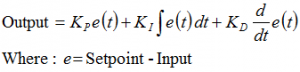This leads pretty much everyone to write the following PID controller:

```/*working variables*/
unsigned long lastTime;
double Input, Output, Setpoint;
double errSum, lastErr;
double kp, ki, kd;
void Compute()
{
/*How long since we last calculated*/
unsigned long now = millis();
double timeChange = (double)(now - lastTime);

/*Compute all the working error variables*/
double error = Setpoint - Input;
errSum += (error * timeChange);
double dErr = (error - lastErr) / timeChange;

/*Compute PID Output*/
Output = kp * error + ki * errSum + kd * dErr;

/*Remember some variables for next time*/
lastErr = error;
lastTime = now;
}

void SetTunings(double Kp, double Ki, double Kd)
{
kp = Kp;
ki = Ki;
kd = Kd;
}
```

Compute() is called either regularly or irregularly, and it works pretty well. This series isn’t about “works pretty well” though. If we’re going to turn this code into something on par with industrial PID controllers, we’ll have to address a few things:

1. Sample Time – The PID algorithm functions best if it is evaluated at a regular interval. If the algorithm is aware of this interval, we can also simplify some of the internal math.
2. Derivative Kick – Not the biggest deal, but easy to get rid of, so we’re going to do just that.
3. On-The-Fly Tuning Changes – A good PID algorithm is one where tuning parameters can be changed without jolting the internal workings.
4. Reset Windup Mitigation –We’ll go into what Reset Windup is, and implement a solution with side benefits
5. On/Off (Auto/Manual) – In most applications, there is a desire to sometimes turn off the PID controller and adjust the output by hand, without the controller interfering
6. Initialization – When the controller first turns on, we want a “bumpless transfer.” That is, we don’t want the output to suddenly jerk to some new value
7. Controller Direction – This last one isn’t a change in the name of robustness per se. it’s designed to ensure that the user enters tuning parameters with the correct sign.
8. NEW: Proportional on Measurement – Adding this feature makes it easier to control certain types of processes

Once we’ve addressed all these issues, we’ll have a solid PID algorithm. We’ll also, not coincidentally, have the code that’s being used in the lastest version of the Arduino PID Library. So whether you’re trying to write your own algorithm, or trying to understand what’s going on inside the PID library, I hope this helps you out. Let’s get started.
Next >>

UPDATE: In all the code examples I’m using doubles. On the Arduino, a double is the same as a float (single precision.) True double precision is WAY overkill for PID. If the language you’re using does true double precision, I’d recommend changing all doubles to floats.### PID Library ported to mbed

Tuesday, July 27th, 2010So the guys over at ARM took the arduino pid library and ported it for use with their mbed platform.Â  Nice little stroke to the ego there.Â  That’s not the main reason I’m excited though.Â Â  Because they’re a legit company,Â  they have written documentation.

I’m terrible at documentation.Â  Commenting my code is hard enough.Â  Writing clear, concise, well-thought-out help files?Â  I’m as useless as 1000 drunk monkeys with typewriters.

It’s always been nagging at me that the Arduino PID Library didn’t have a clear help document. Now, indirectly, it does. Since the backend is the same, everything they say about the mbed library works with the Arduino library as well.

### PID Front-End v0.2

Saturday, July 4th, 2009Some people spend the 4th of July relaxing with friends, drinking beer, enjoying some good weather. I… updated the pid front-end. I got several requests to add grid lines and axes, and now was the time. For those of you with an image of me in a dank basement coding away, fear not. There was a Hawaiian shirt and a hammock involved.

The picture says it all. The trend lines are a little thicker, and there are now axis labels and grid lines. It’s also worth noting, since it was a pain to code, that the time gridlines and labels scroll, and can be displayed in milliseconds, seconds or minutes.

### Arduino Reflow Toaster

Sunday, June 14th, 2009Pic 1: Arduino Controlled Toaster-Oven. No, I have not tried making toast with it

The Mark 1 reflow toaster is complete! (“Mark 1” is another way of saying there’s a lot of work to be done.)

It can hold +/- 1 Â°F, which should be sufficient for surface mount electronics. I say “should be” because I haven’t soldered anything with it yet.

### Construction:Pic 2: Termistor support mast

Temperature sensing is done by a thermistor. Simple solder connections and 20ga wire get the signal back to the Arduino. (UPDATE: the thermistor is wired at the Arduino using this method.) The wires are run through a copper tube, and everything is held in place with JB weld. This setup seems to work, even at temperatures that melt solder. I don’t know if that’s because the JB keeps the solder connections from melting, or if it holds the molten solder in place during run-time. Either way, I haven’t had any problems getting temperature readings.

On the heat side, an SSR is spliced directly into the 120V plug wire. This is not safe. The SSR is fine, but having exposed 120V dangling near the toaster is a recipe for bad times. In the mark 2 that will all be neatly enclosed.

The toaster’s intrinsic temperature control was left in place, turned all the way up to 500Â°F.Â  If everything is working correctly, it will stay out of the way.Â  If something goes wrong, the temperature will be prevented from rising without bound.

### Temperature Control:

I decided to use the Arduino PID Library to control the oven temperature. I think it works really well, but I’m a little biased.Pic 3: Temperature control: On-Off vs PID. On-Off is easier, while PID provides tighter control with a more stable output

The easiest way to control temperature would be to use on-off control: “If I’m too cold, turn on. if I’m too hot, turn off.” I tried that first. As you can see in the picture above, it does a decent job. The oven temperature (red line) stays within 5 Â°F of where we want to be (green line.) This is probably fine in this application. The one questionable thing is the output. Since it’s cycling between full on and full off, there might be some extra thermal shock to the system.

On-Off control is probably fine, but as I said, I’m biased. I decided to implement pid control. As you can see, it does a better job of staying where we want to be. Instead of 5 degrees, now the temperature stays within 1 degree. Also, since the output is essentially constant, there’s a better chance that there will be a uniform temperature gradient in the oven.

The extra effort probably wasn’t necessary, but I still think it’s pretty cool.

### Next Steps

• I think the termistor may be in a bad location.Â  I wanted it to be really close to the workpiece, so I put it directly underneath where the workpiece would go.Â  it seems that when there’s a piece in place, it actually changes how heat flows in that area, and the temperature reading is almost 20 degrees higher.Â  I’ll need to see if this adversely impacts reflow…Â  once I actually reflow something.
• I may need to upgrade to a convection toaster to get more uniform temperature distribution
• currently I’m adjusting setpoints and watching progress using the PID frontend.Â  it would be nice to move that functionality onto the arduino. maybe an LCD and some buttons…
• It might be cool to have a pre-programmed setpoint profile that the oven can cycle through

### Graphical Front-End for the Arduino PID Library

Saturday, May 30th, 2009The first (extreme beta) version of the PID Front-End is up on the pid library page. It makes adjusting an Arduino PID much, much easier. The download includes the processing sketch (of course.) It also has an Arduino sketch showing how to send/receive on that end.